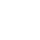WELCOME TO TIANJIN HIGHLEM TECH. CO., LTD.

Industrial Solutions

## Accumulator Applications

1. 选择方法

1) 最小压力P1和最大压力P2－工作压力。

2) 可储存或利用的液体容积△V .

3) 方法和用途

4) 工作温度

5) 液体种类

6) 所需大流量

7) 使用场所

2.预充气压力

Po的极限值为：Po=0.9P1  Po min≥0.25×P2  Po max≤0.9P1

Ø 活塞蓄能器  Po=0.95-0.97 P1

Ø 脉动缓冲和减振Po=0.6-0.75 Pm或Po=0.8P1

Ø 液路缓冲

Po=0.6-0.9Pm

Ø 蓄能器+附加气瓶

Po=0.95-0.97P1

Po值适用于用户所要求的最大工作温度。

Poc=Po3．计算原理

Po×Von=P1×V1n= P2×V2n

V1=压力P1时的氮气体积 P1（升）

V2=压力P2时的氮气体积 P2（升）

△V=所排放的或所储存的液体的容积（升）

Po=预充氮气压力（巴）

P1=最小工作压力（巴）

P2=最大工作压力（巴）

n =多变指数

n = 1 如果氮气的压缩和膨胀发生的很慢，使得整个热交换过程位于气体和周围介质之间，这就是在恒温下；该状态为等温。

n = 1.4 如果运行速度进行的非常快，使得热交换没有发生；该状态为绝热。4 容积计算（等温）

Po×Vo=P1×V1= P2×V2

△V=V1－V2=Vo－VoVo=5 容积补偿（等温）

△V= VT×（T2－T1）×（β－3α）

VT =管道容积（升）

T2 =最大温度（℃）

T1 =最小温度（℃）

β= 流体的体积膨胀系数（1/℃）

α= 管道线性膨胀系数（1/℃）

P1=最小允许工作压力（bar）

P2=最大允许工作压力（bar）

Vo=6 泄漏补偿（等温）

ΔV=Q1×t

Po=0.9×P1

P1=最小允许工作压力（bar）

P2=最大允许工作压力（bar）

Vo =7 容积计算（绝热）

Po×Von=P1×V1n= P2×V2n

ΔV = Vo=0.7143

Vo =VOT=VO；T2=t2(℃)+273=最大工作温度（°K）

T1=t1(℃)+273=最小工作温度（°K）

VO=忽略了温度变化而计算出的容积（升）

VOT=考虑温度变化后的实际容积（升）

VOT=VO×Ci×（等温）

VOT=VO×Ca×（绝热）8紧急能量存储

Vo =ΔV = Von =1.4 绝热系数（快速排放阶段）

nc = 1-1.4多变系数（慢速排放阶段）

9 吸收脉动Vo =q=泵的工作容积（升）

=A×C(活塞面积×冲程)=Q/N（流率/冲程）

P=泵的平均工作压力（bar）

P1=P-X(bar)

P2=P+X(bar)

α=剩余脉动±（%）

K=此为系数，它与活塞的数量及泵的作用方式有关。

泵型号        K

1只活塞，单作用式  0.69

1只活塞，双作用式  0.29

2只活塞，单作用式  0.29

2只活塞，双作用式  0.17

3只活塞，单作用式  0.12

3只活塞，双作用式  0.07

4只活塞，单作用式  0.13

4只活塞，双作用式  0.07

5只活塞，单作用式  0.07

5只活塞，双作用式  0.023

6只活塞，单作用式  0.07

7只活塞，双作用式  0.023

10. 液压管线吸收冲击

ΔPmax(bar) =Vo=Vo= 蓄能器的容积 (升)

Q = 管路中的流量 (m3／h)

L = 管路的总长度（m）

γ = 液体的比重（Kg／m3）

V = 流速 (m/s)  =1000Q／3.6S

S = 管路的内截面积 (mm2)   =0.25×πd2

d = 管路内径(mm)

ΔP= 允许的超压(bar)

P1 = 自由流动的工作压力 (绝对压力，bar)

P2 = 允许的最大压力 (绝对压力，bar)  = P1+ΔP

t = 减速时间(阀门关闭时间) (s)

11. 蓄能器＋附加气瓶（传送）

VOB =VOT －VOA

VOA = 蓄能器的容积

VOB = 附加气瓶的容积

Email: hailaimu@high-lem.com highlem@163.com Telephone: 18222914688

Hellem company is founded by the accumulator industry technical expert team, has accumulated rich accumulator, release agent spraying experience for more than 30 years, has 34 independent intellectual property rights of various products,Is the accumulator products and application solutions provider of the capsule diaphragm piston hydraulic industry, the products meet the quality and safety standards of various countries.